## If you wish to take a picture of a bullet traveling at 500 m/s, then a very brief flash of light produced by an RC discharge through a flash

Question

If you wish to take a picture of a bullet traveling at 500 m/s, then a very brief flash of light produced by an RC discharge through a flash tube can limit blurring. Assuming 1.00 mm of motion during one RC constant is acceptable, and given that the flash is driven by a 600-μF capacitor, what is the resistance in the flash tube?

in progress 0
2 weeks 2021-09-04T11:24:17+00:00 1 Answers 0 views 0

1. Explanation:

The given data is as follows.

Speed of the bullet (v) = 500 m/s

Distance during one RC time constant (d) = 1 mm =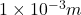Capacitance (C) = 600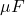=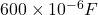Hence, formula for speed of the bullet is as follows.

v =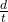or,           t =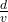Time constant for RC circuit is as follows.

t = RC

R =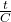=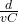=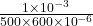=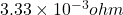Thus, we can conclude that resistance in the flash tube is.